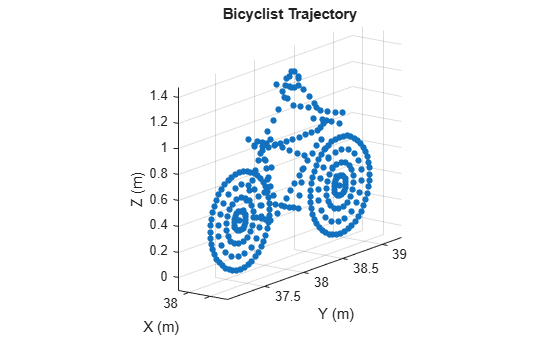# move

Position, velocity, and orientation of moving bicyclist

## Syntax

``[bpos,bvel,bax] = move(bicyclist,T,angh)``
``[bpos,bvel,bax] = move(bicyclist,T,angh,speed)``
``[bpos,bvel,bax] = move(bicyclist,T,angh,speed,coast)``

## Description

example

````[bpos,bvel,bax] = move(bicyclist,T,angh)` returns the current positions, `bpos`, and current velocities, `bvel`, of the scatterers and the current orientation axes, `bax`, of the bicyclist. The positions, velocities, and axes are then updated for the next time interval `T`. `angh` specifies the heading angle of the bicyclist.```

example

````[bpos,bvel,bax] = move(bicyclist,T,angh,speed)` also specifies the `speed` of the bicyclist.```
````[bpos,bvel,bax] = move(bicyclist,T,angh,speed,coast)` also specifies the coasting state, `coast`, of the bicyclist.```

## Examples

collapse all

Plot the positions of all bicyclist scatterers. Assume there are 15 spokes per wheel.

Create a `backscatterBicyclist` object for a radar system operating at 77 GHz and having a bandwidth of 300 MHz. The sampling rate is twice the bandwidth. The bicyclist is initially 5 meters away from the radar.

```bw = 300e6; fs = 2*bw; fc = 77e9; rpos = [0;0;0]; bpos = [5;0;0]; bicyclist = backscatterBicyclist( ... 'OperatingFrequency',fc,'NumWheelSpokes',15, ... 'InitialPosition',bpos);```

Obtain the initial position of the scatterers and advance the motion by 1 second.

`[bpos,bvel,bax] = move(bicyclist,1,0);`

Obtain the number of scatterers and the indices of the wheel scatterers.

```N = getNumScatterers(bicyclist); Nsw = (N-114+1)/2; idxfrontwheel = (114:(114 + Nsw - 1)); idxrearwheel = (114 + Nsw):N;```

Plot the locations of the scatterers.

```plot3(bpos(1,1:90),bpos(2,1:90),bpos(3,1:90), ... 'LineStyle','none','Color',[0.5,0,0],'Marker','.') axis equal hold on plot3(bpos(1,91:99),bpos(2,91:99),bpos(3,91:99), ... 'LineStyle','none','Color',[0,0,0.7],'Marker','.') plot3(bpos(1,100:113),bpos(2,100:113),bpos(3,100:113), ... 'LineStyle','none','Color',[0,0,0],'Marker','.') plot3(bpos(1,idxfrontwheel),bpos(2,idxfrontwheel),bpos(3,idxfrontwheel), ... 'LineStyle','none','Color',[0,0.5,0],'Marker','.') plot3(bpos(1,idxrearwheel),bpos(2,idxrearwheel),bpos(3,idxrearwheel), ... 'LineStyle','none','Color',[0.5,0.5,0.5],'Marker','.') hold off legend('Frame and rider','Pedals','Rider legs','Front wheel','Rear wheel')```Display an animation of a bicyclist riding in a quarter circle. Use the default property values of the `backscatterBicyclist` object. The motion is updated at 30 millisecond intervals for 500 steps.

```dt = 0.03; M = 500; angstep = 90/M; bicycle = backscatterBicyclist; for m = 1:M [bpos,bvel,bang] = move(bicycle,dt,angstep*m); plot(bicycle) end```## Input Arguments

collapse all

Bicyclist, specified as a `backscatterBicyclist` object.

Duration of next motion interval, specified as a positive scalar. The scatterer positions and velocities and bicyclist orientation are updated over this time duration. Units are in seconds.

Example: `0.75`

Data Types: `double`

Heading of the bicyclist, specified as a scalar. Heading is measured in the xy-plane from the x-axis towards the y-axis. Units are in degrees.

Example: `-34`

Data Types: `double`

Bicyclist speed, specified as a nonnegative scalar. The motion model limits the speed to 60 m/s. Units are in meters per second. Alternatively, you can specify the bicyclist speed using the `Speed` property of the `backscatterBicyclist` object.

Example: `8`

Data Types: `double`

Set bicyclist coasting state, specified as `false` or `true`. If set to `true`, the bicyclist is not pedaling, but the wheels are still rotating (freewheeling). If set to `false`, the bicyclist is pedaling, and the `GearTransmissionRatio` determines the ratio of wheel rotations to pedal rotations. Alternatively, you can specify the bicyclist coasting state using the `Coast` property of the `backscatterBicyclist` object.

Data Types: `logical`

## Output Arguments

collapse all

Positions of bicyclist scatterers, returned as a real-valued 3-by-N matrix. Each column represents the Cartesian position, [x;y;z], of one of the bicyclist scatterers. N represents the number of scatterers and can be obtained using the `getNumScatterers` object function. Units are in meters. See Bicycle Scatterer Indices for the column representing the position of each scatterer.

Data Types: `double`

Velocities of bicyclist scatterers, returned as a real-valued 3-by-N matrix. Each column represents the Cartesian velocity, [vx;vy;vz], of one of the bicyclist scatterers. N represents the number of scatterers and can be obtained using the `getNumScatterers` object function. Units are in meters per second. See Bicycle Scatterer Indicesfor the column representing the velocity of each scatterer.

Data Types: `double`

Orientation axes of bicyclist, returned as a real-valued 3-by-3 matrix. Units are dimensionless.

Data Types: `double`

collapse all

### Bicycle Scatterer Indices

Bicyclist scatterer indices define which columns in the scatterer position or velocity matrices contain the position and velocity data for a specific scatterer. For example, column 92 of `bpos` specifies the 3-D position of one of the scatterers on a pedal.

The wheel scatterers are equally divided between the wheels. You can determine the total number of wheel scatterers, N, by subtracting 113 from the output of the `getNumScatterers` function. The number of scatterers per wheel is Nsw = N/2.

Bicyclist Scatterer Indices

Bicyclist ComponentBicyclist Scatterer Index
Frame and rider1 … 90
Pedals91 … 99
Rider legs100 … 113
Front wheel114 … 114 + Nsw - 1
Rear wheel114 + Nsw … 114 + N - 1

## Extended Capabilities

### C/C++ Code GenerationGenerate C and C++ code using MATLAB® Coder™.